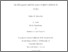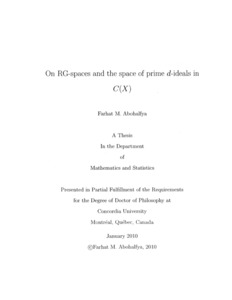Title:

# On RG-spaces and the space of prime d-ideals in C(X)

Abohalfya, Farhat M (2010) On RG-spaces and the space of prime d-ideals in C(X). PhD thesis, Concordia University.Preview Text (application/pdf) NR71154.pdf - Accepted Version 3MB

## Abstract

Let A be a commutative semiprime ring with identity. Then A has at least two epimorphic regular extensions namely, the universal epimorphic regular extension T (A ), and the epimorphic hull H (A ). We are mainly interested in the case of C(X) , the ring of real-valued continuous functions defined on a Tychonoff space X . It is a commutative semiprime ring with identity and it has another important epimorphic regular extension namely, the minimal regular extension G ( X ). In our study we show in chapter 5 that the spectrum of the ring H (A ) with the spectral topology is homeomorphic to the space of the prime i-ideals in A with the patch topology. In the case of C(X) , the spectrum of the epimorphic hull H(X) with the spectral topology is homeomorphic to the space of prime d -ideals in C(X) with the patch topology. A Tychonoff space X which satisfies the property that G (X ) = C ( X e ) is called an RG-space. We shall introduce a new class of topological spaces namely the class of almost k -Baire spaces, and as a special case of this class we shall have the class of almost Baire spaces. We show that every RG-space is an almost Baire space but it need not be a Baire space. However in the case of RG-spaces of countable pseudocharacter, RG-spaces have to be Baire spaces. Furthermore in this case every dense set in RG-spaces has a dense interior. The Krull z -dimension and the Krull d -dimension will play an important role to determine which of the extensions H(X) and G (X ) has the form of a ring of real-valued continuous functions on some topological space. In  the authors gave some techniques to prove that there is no RG-space with infinite Krull z-dimension, but there was an error that we found in the proof of theorem 3.4. In this study we will give an accurate proof which applies to many spaces but the general theorem will remain open. And we will use the same techniques to prove that if C(X) has an infinite chain of prime d -ideals then H(X) cannot be isomorphic to a ring of real-valued continuous functions

Divisions: Concordia University > Faculty of Arts and Science > Mathematics and Statistics Thesis (PhD) Abohalfya, Farhat M vii, 102 leaves : ill. ; 29 cm. Concordia University Ph. D. Mathematics 2010 Raphael, Robert LE 3 C66M38P 2010 A36 979428 Concordia University Library 09 Dec 2014 17:59 13 Jul 2020 20:12 https://concordiauniversity.on.worldcat....
All items in Spectrum are protected by copyright, with all rights reserved. The use of items is governed by Spectrum's terms of access.

Repository Staff Only: item control pageResearch related to the current document (at the CORE website)
Back to top